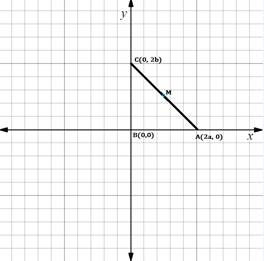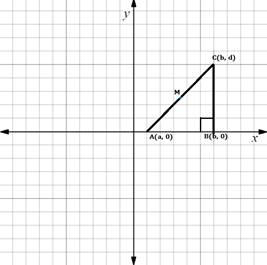Chapter 10.CT, Problem 14CTElementary Geometry For College St...

7th Edition
Alexander + 2 others
ISBN: 9781337614085

Solutions

Chapter
SectionElementary Geometry For College St...

7th Edition
Alexander + 2 others
ISBN: 9781337614085
Textbook Problem

For providing the theorem “The midpoint of the hypotenuse of a right triangle is equidistant from all three vertices.” which drawing is best? a)b)c)To determine

(a)

Which is the best drawing for the given theorem: “The midpoint of the hypotenuse of a right triangle is equidistant from all three vertices.”

Explanation

Given theorem,

The midpoint of the hypotenuse of a right triangle is equidistant from all three vertices

Given drawing,

ABC is the triangle, where

Midpoint of CA is M =0+02,0+

To determine

(b)

Which is the best drawing for the given theorem: “The midpoint of the hypotenuse of a right triangle is equidistant from all three vertices.”

To determine

(c)

Which is the best drawing for the given theorem: “The midpoint of the hypotenuse of a right triangle is equidistant from all three vertices.”

Still sussing out bartleby?

Check out a sample textbook solution.

See a sample solution

The Solution to Your Study Problems

Bartleby provides explanations to thousands of textbook problems written by our experts, many with advanced degrees!

Get Started

Change each percent to a decimal: 814

Elementary Technical Mathematics

83-94 Evaluate the integral. 55edx

Calculus (MindTap Course List)

Sketch a graph of each of the following functions.

Mathematical Applications for the Management, Life, and Social Sciences

Minimize subject to C=24x+18y+24z 3x+2y+z4x+y+3z6x0,y0,z0

Finite Mathematics for the Managerial, Life, and Social Sciences

True or False: f(a)=lima0f(a+h)f(a)h.

Study Guide for Stewart's Single Variable Calculus: Early Transcendentals, 8th